Courses

# Henry’s Law Civil Engineering (CE) Notes | EduRev

## Civil Engineering (CE) : Henry’s Law Civil Engineering (CE) Notes | EduRev

The document Henry’s Law Civil Engineering (CE) Notes | EduRev is a part of the Civil Engineering (CE) Course Thermodynamics.
All you need of Civil Engineering (CE) at this link: Civil Engineering (CE)

Henry’s Law

The solubility of gases that are sparingly soluble in solvents constitutes a special application of the general VLE relations developed in sections 7.3 and 7.4. There are numerous real-life examples of such situations; for example, the solubilization of oxygen in water, which sustains aqueous life. Similarly, gases such as nitrogen, carbon dioxide, etc., display relatively low solubility (mole fraction: 10−5 − 10−2 ) in water or many solvents of industrial interest.  Further, in many such instances, the solubility of a gas in a solvent is required at temperatures beyond the critical temperature of the gas.
Application of vapour-liquid phase equilibria relations given by Raoult's law or its modified versions (discussed in the foregoing sections) to a solute species i (in a solvent) requires the saturation vapour pressure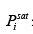at the temperature of application. Clearly if the temperature of interest exceeds the critical temperature of the solute, the parameter Pi sat is not definable, and hence such VLE relations presented in sections 7.5 and 7.6 are not appropriate in such cases. As for any VLE problem the starting point for determining the solubility of a gaseous species ‘i’ in a liquid is the equality of the fugacity of the solute species and liquid (liq) phases: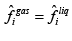.....(7.81)
Using eqn. 7.45 (considering low to moderate pressures):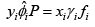.....(7.45)

Denoting the gaseous solute as ‘1’ and the solvent as ‘2’, one may write: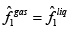.....(7.82)

And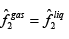.....(7.83)

Using eqn. 7.45 the last two equations may be re-written as: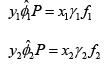.....(7.84 & 7.85)

If we further assume that the gas is very sparingly soluble in the solvent, the liquid phase is essentially pure solvent and the following relations derive: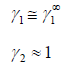Therefore, for component 1 we may rewrite the eqn. 7.84 as: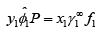.....(7.86)
Or alternately: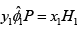.....(7.87)

Where: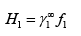.....(7.88)

Equation 7.88 is termed the Henry’s law, and H1 the Henry’s constant, which is defined at the system temperature. If one plots the value of fˆ1 as a function of the gas mole fraction x1 in the solvent phase (as shown schematically in fig. 7.13), the parameter H1 corresponds to the slope of the tangent drawn on the curve at the limiting condition of x1 → 0.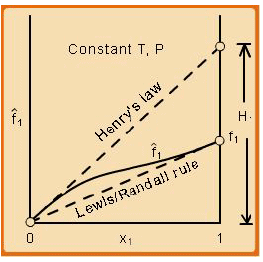Fig. 7.13 Plot of fˆ1 as a function of the gas mole fraction x1

Similarly for component 2 the phase equilibrium equation 7.85 may be rewritten as: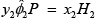.....(7.89)

Where: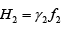.....(7.90)

Since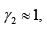it follows that:

Where: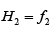.....(7.91)

Thus: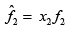.....(7.92)

It may be noted that eqn. 7.92 is the same as 6.162 (section 6.15), which describes the Lewis -Randall rule. Thus when Henry’s law is applicable for the solute then Lewis-Randall rule is applicable for the solvent. Since for a system temperature T >Tc,1 the fugacity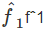of pure liquid phase for ‘1’ is hypothetical, it follows that the Henry’s law constant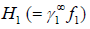is necessarily a hypothetical quantity as well. Since solubility of a gas is temperature dependent, it follows that H i is also a function of temperature. The Henry’s law constant for a large number of gases with water as the solvent has been reported in the literature. For example for acetylene the value is 1350bar, for carbon dioxide 1670bar, and for air 72950bar). Fig. 7.14 presents the value of Henry’s law constant for a number of gases in water as a function of temperature.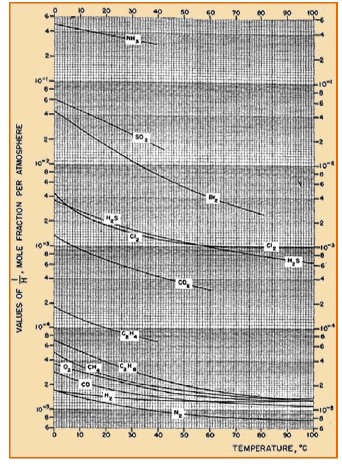Fig. 7.14 Plot of Henry’s Constant vs. Temperature, [(1 H ) = N (mole fraction) P(atm)]

Offer running on EduRev: Apply code STAYHOME200 to get INR 200 off on our premium plan EduRev Infinity!

## Thermodynamics

56 videos|92 docs|33 tests

,

,

,

,

,

,

,

,

,

,

,

,

,

,

,

,

,

,

,

,

,

;